Function Repository Resource:

# BezoutMatrix

Generate the Bézout matrix of two univariate polynomials

Contributed by: Jan Mangaldan
 ResourceFunction["BezoutMatrix"][poly1,poly2,var] returns the Bézout matrix of the polynomials poly1 and poly2 with respect to the variable var.

## Details

The Bézout matrix is symmetric and has dimensions Max[m,n]×Max[m,n], where m and n are respectively the degrees of poly1 and poly2.
The determinant of the Bézout matrix of two polynomials is proportional to their resultant.

## Examples

### Basic Examples (1)

The Bézout matrix of two polynomials:

 In:=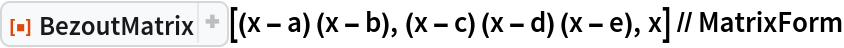Out=### Scope (2)

The Bézout matrix of polynomials with numeric coefficients:

 In:=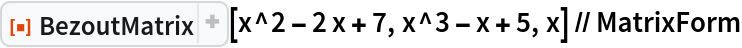Out=The Bézout matrix of polynomials with parametric coefficients:

 In:=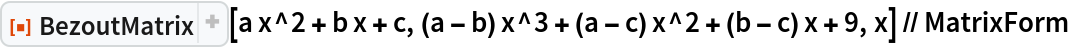Out=### Applications (2)

A polynomial is stable if all of its roots have negative real parts. Use BezoutMatrix to check the stability of a polynomial:

 In:=Out=In:=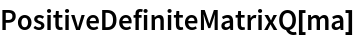Out=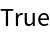Verify stability by computing the roots of the polynomial:

 In:=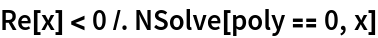Out=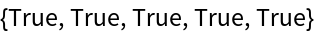### Properties and Relations (2)

The Bézout matrix is symmetric:

 In:=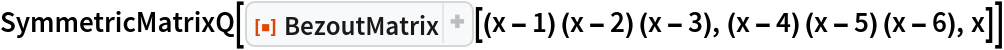Out=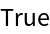The determinant of the Bézout matrix of two polynomials is proportional to their resultant:

 In:=In:=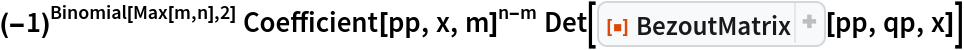Out=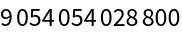In:=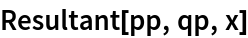Out=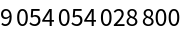### Neat Examples (1)

Verify a relationship between the Bézout matrix and the Sylvester matrix:

 In:=In:=In:=In:=Out=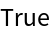## Version History

• 1.0.0 – 01 March 2021